• 余弦相似度
2022-07-12 11:12:07

目录

一、基本算法--余弦相似度

二、计算余弦相似度

2.1、python实现

2.2、sklearn

2.3、scipy  ---最快

2.4、pytorch

三、参考

## 一、基本算法--余弦相似度

1、使用TF-IDF算法，找出两篇文章的关键词；
2、每篇文章各取出若干个关键词（比如20个），合并成一个集合，计算每篇文章对于这个集合中的词的词频TF（为了避免文章长度的差异，可以使用相对词频）；
3、生成两篇文章各自的词频向量；
4、计算两个向量的余弦相似度，值越大就表示越相似
假设向量a、b的坐标分别为(x1,y1)、(x2,y2) 。则：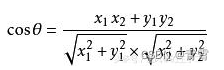设向量 A = (A1,A2,...,An)，B = (B1,B2,...,Bn) 。推广到多维，数学家已经帮我们证明了，所以你只要记住下面的公式：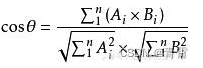简单来说可以写成下面的式子：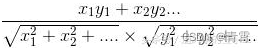举一个具体例子，我们先从句子开始：
句子A：我喜欢看电视，不喜欢看电影。
句子B：我不喜欢看电视，也不喜欢看电影。
第一步：分词
句子A：我/喜欢/看/电视，不/喜欢/看/电影。
句子B：我/不/喜欢/看/电视，也/不/喜欢/看/电影。
第二步：列出所有的词
我，喜欢，看，电视，电影，不，也
第三步：计算词频
句子A：我 1，喜欢 2，看 2，电视 1，电影 1，不 1，也 0
句子B：我 1，喜欢 2，看 2，电视 1，电影 1，不 2，也 1
第四步：写出词频向量
句子A：[1, 2, 2, 1, 1, 1, 0]
句子B：[1, 2, 2, 1, 1, 2, 1]
第五步：计算余弦值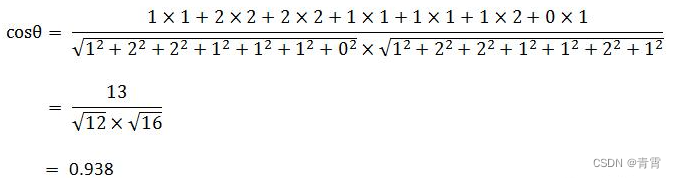余弦值越接近1，就表明夹角越接近0度，也就是两个向量越相似，这就叫"余弦相似性"
简单来说上面计算出的值代表两个句子大概九成相似，越接近1就越相似。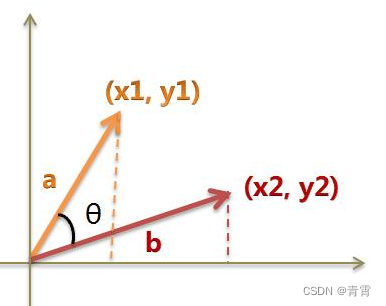## 二、计算余弦相似度

余弦相似度(值越大，两者越相似，向量夹角越小，极限是重合，夹角为0，此时余弦相似度是1）。

### 2.1、python实现

自己实现一个，按照cos的计算公式——向量的内积除以向量模长的积代码如下：def python_cos(q_vec, b_vec):
"""
计算余弦相似度
:param q_vec: 一维数组
:param b_vec: 一维数组
:return:
"""
dot_q_b = 0
q_vec_length = 0
b_vec_length = 0
for q, b in zip(q_vec, b_vec):
dot_q_b += q * b
q_vec_length += q * q
b_vec_length += b * b
length = (q_vec_length ** (1 / 2)) * (b_vec_length ** (1 / 2))
cos_sim = dot_q_b / length  # 向量的内积除以向量模长的积
return cos_sim

a = [1, 2, 2, 1, 1, 1, 0]
b = [1, 2, 2, 1, 1, 2, 1]
print(python_cos(a, b))   # cos_sim 0.9381941874331419。

### 2.2、sklearn

sklearn机器学习算法常用库，这个就比较简单了只需要直接调用sklearn中相关的API即可：
pip3 install -U scikit-learn
from sklearn.metrics.pairwise import cosine_similarity

def cos(a, b):
"""
a和b都要是2维
"""
cos = cosine_similarity(a,b)
return cos

a = [[1,2,3],[3,2,1], [3,4,5]]
b = [[3,2,1],[1,2,3]]
print(cos(a, b))

'''结果    a中的每一项与b中每一项的相似度
[[0.71428571  1.        ]
[1.          0.71428571]
[0.83152184  0.98270763]]
'''

注意这里的参数，a和b都必须是二维的，不然会报错；a和b中的元素个数不定。

### 2.3、scipy  ---最快

import scipy.spatial

@coast_time
def cos(a, b):
'''
a和b都要是2维,a和b中的元素个数不定
'''
dis = scipy.spatial.distance.cdist(a,b,'cosine')
cos = 1 - dis
return cos

a = [[1,2,3]]
b = [[3,2,1],[4,5,6], [2,2,3]]
print(cos(a, b))
'''结果
func cos coast time:0.00006230 s
[0.71428571 0.97463185 0.97230559]
'''

注意这里的参数，a和b的数据类型可以是数组、np.array();a和b都必须是二维的；a和b中的元素个数不定。这里dis可以理解为余弦距离，要用1-dis才是余弦相似度。

### 2.4、pytorch

sklearn机器学习算法常用库，这个就比较简单了只需要直接调用sklearn中相关的API即可：
PyTorch  windows安装torch： pip3 install torch torchvision torchaudio
import torch
torch.set_default_tensor_type(torch.FloatTensor)

@coast_time
def torch_cos_new(a, b):
"""
a,b必须是tensor张量；torch_cos中有个bug，a和b的第一维只能是1，不然会出现错误结果
"""
cos = torch.cosine_similarity(a, b, dim=1)
return cos

a = torch.tensor([[1,2,3]]).float()  # 转化为tensor张量
b = torch.tensor([[3,2,1],[4,5,6], [2,2,3]]).float()
print(torch_cos_new(a, b))

'''结果
tensor([0.7143, 0.9746, 0.9723])
func torch_cos_new coast time:0.00072610 s
'''

## 三、参考

https://www.haitaoseo.com/950191.html  文章相似度检测工具（一种简单高效的算法）

https://github.com/zhao-dapeng/Cosine   zhao-dapeng/Cosine

余弦相似度计算的实现方式_colourmind的博客-CSDN博客_余弦相似度计算代码余弦相似度
更多相关内容
• 余弦相似度计算matlab代码计算余弦相似度 张伯超 该脚本将从免疫数据库中读取数据并计算不同特征样本之间的余弦相似度。 用法 -d name of database -s name of subject -f field of the columns used to separate ...
• 火花余弦相似度这是一个脚本，输入一个矩阵并计算矩阵中每个向量与其他向量的余弦相似度例子： *add test dataset (dataset.txt) into hadoop hdfs这是数据集的摘录： "16",45,12,7,2,2,2,2,4,7,7"28",1,1,1,0,0,0,0...Python
• Matlab余弦相似度算法判断图片相似度并识别源代码 Matlab 余弦相似度 图像匹配 可直接运行 Matlab余弦相似度算法判断图片相似度并识别源代码 Matlab 余弦相似度 图像匹配 可直接运行
• StaySense-快速余弦相似度ElasticSearch插件使用向量嵌入在ElasticSearch 6.4.x +上实现极快的向量评分。 关于StaySense ： 是一家革命性的软件公司，创建了有史以来最先进的营销软件，可供度假租赁和酒店行业的酒店...Java
• （python）使用余弦相似度算法计算两个文本的相似度的简单实现
• 也可以叫余弦相似度。 几何中夹角余弦可用来衡量两个向量方向的差异，机器学习中借用这一概念来衡量样本向量之间的差异。 (1)在二维空间中向量A(x1,y1)与向量B(x2,y2)的夹角余弦公式： (2) 两个n维样本点a(x11,x12,...
• 余弦相似度，又称为余弦相似性，是通过计算两个向量的夹角余弦值， 来评估他们的相似度。 余弦相似度将向量根据坐标值，绘制到向量空间中，如常见的二维空间。 余弦相似度衡量的是2个向量间的夹角大小，通过夹角的...
• 衡量两个向量x、y的相关性(夹角的余弦) MATLAB 代码 ，余弦相似度也被⼀些迁移学习研究所使用。
• Java 实现推荐系统 两个字符串 余弦相似度 算法。
• 提取任何图像的特征向量，并找到余弦相似度以使用Pytorch进行比较。 我已经使用ResNet-18提取图像的特征向量。 最后，开发了一个Django应用程序来输入两个图像并找到余弦相似度。 包装方式： 火炬 Django 2.0 学分...
• Go (Golang) 中的余弦相似度 返回 2 []float64 的相似度 (float64)Go
• 实现一个电影推荐系统，采用协同过滤算法，相似度算法为余弦相似度，基于用户和基于项目中选择基于项目数据集为movielens数据集 一，项目说明 项目名称：item_cf_go 语言：golang 项目地址：github....Go
• 给出了一种基于属性熵和加权余弦相似度的离群数据挖掘算法LEAWCD．该算法首先根据局部属性熵分析每个对象在其k．邻域内的局部离群属性，并依据各离群属性的属性偏离度自动设置属性权向量；其次使用对高维数据有效的...
• 两个给定文本之间的余弦相似度 在终端上运行程序： $javac CosineSimilarity.java$ java CosineSimilarity.java 在此之后，输入两个字符串文本。 程序将生成向量映射表，然后是文本之间的余弦相似度得分。Java
• 该插件不再维护使用此插件，您可以使用点积或余弦相似度，根据任意原始向量对文档进行评分。发行版Master分支的目标是Elasticsearch 5.4。 请注意，不支持5.5+版本，因为Elasticsearch更改了其插件机制。 5.5+的...Java
• 基于内容推荐算法和余弦相似度算法的领导决策辅助信息系统.pdf
• 称呼： Cs = getCosineSimilarity（x，y） 计算向量 x 和 y 之间的余弦相似度。 x和y必须具有相同的长度。 的解释余弦相似度类似于皮尔逊相关matlab
• 余弦相似度和欧氏距离Photo by Markus Winkler on Unsplash Markus Winkler在Unsplash上拍摄的照片 This is a quick and straight to the point introduction to Euclidean distance and cosine similarity with a ...

余弦相似度和欧氏距离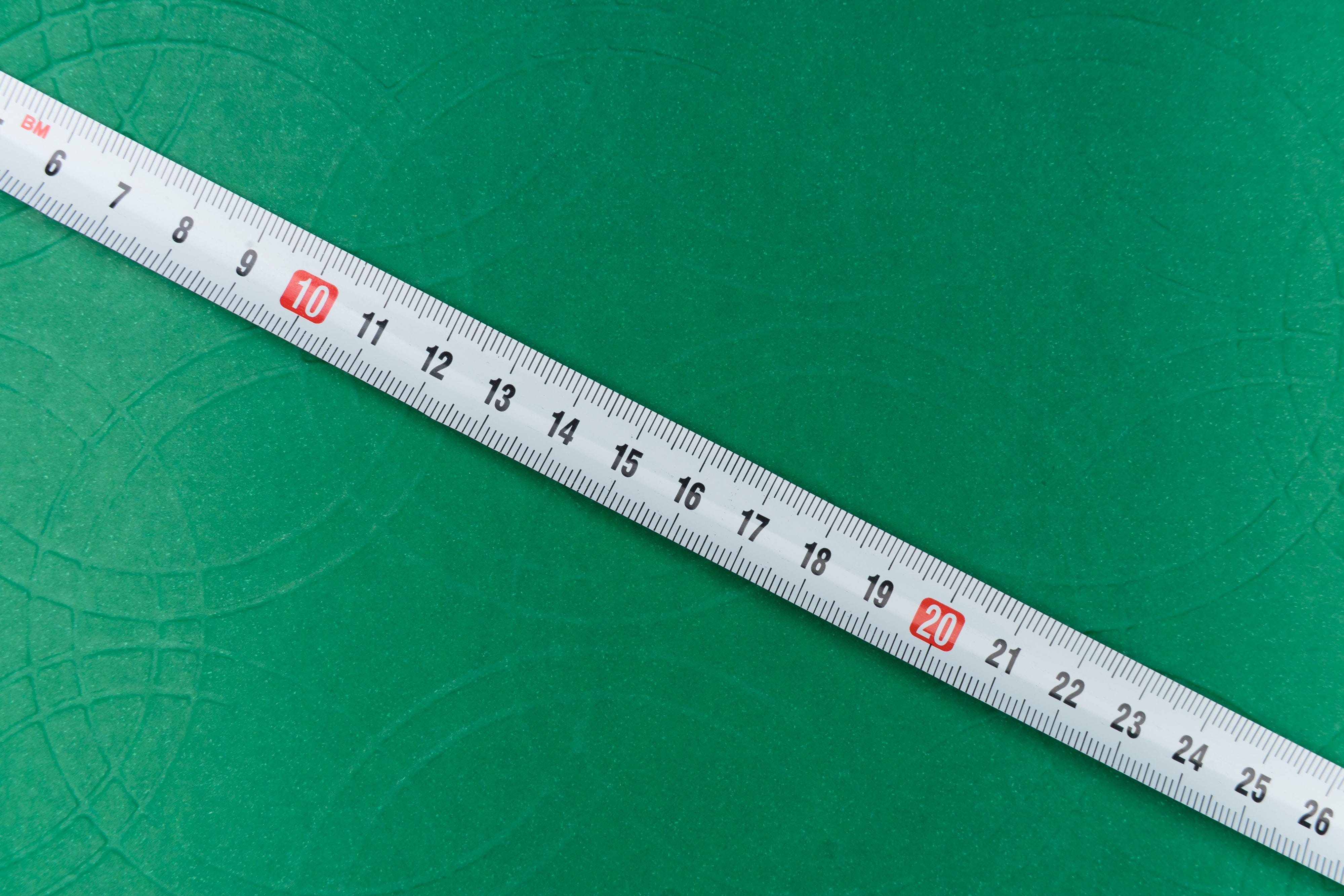Photo by Markus Winkler on Unsplash

This is a quick and straight to the point introduction to Euclidean distance and cosine similarity with a focus on NLP.

这是对欧氏距离和余弦相似度的快速而直接的介绍，重点是NLP。

# 欧氏距离 (Euclidean Distance)

The Euclidean distance metric allows you to identify how far two points or two vectors are apart from each other.

欧几里德距离度量标准可让您确定两个点或两个向量彼此相距多远。

Now suppose you are a high school student and you have three classes. A math class, a philosophy class, and a psychology class. You want to check the similarity between these classes based on the words your professors use in class. For the sake of simplicity, let’s consider these two words: “theory” and “harmony”. You could then create a table like this to record the occurrence of these words in each class:

现在假设您是一名高中生，您有3个班级。 数学课，哲学课和心理学课。 您想根据您的教授在课堂上使用的单词来检查这些课程之间的相似性。 为了简单起见，让我们考虑以下两个词：“理论”和“和谐”。 然后，您可以创建一个像这样的表来记录每个类中这些单词的出现情况：

In this table, the word “theory” is repeated 60 times in math class, 20 times in philosophy class, and 25 times in psychology class whereas the word harmony is repeated 10, 40, and 70 times in math, philosophy, and psychology classes respectively. Let’s translate this data into a 2D plane.

在此表中，“理论”一词在数学课中重复了60次，在哲学课中重复了20次，在心理学课中重复了25次，而在数学，哲学和心理学课中，“和谐”一词重复了10、40和70次分别。 让我们将此数据转换为2D平面。

The Euclidean distance is simply the distance between the points. In the graph below.

欧几里得距离就是点之间的距离。 在下图中。

You can see clearly that d1 which is the distance between psychology and philosophy is smaller than d2 which is the distance between philosophy and math. But how do you calculate d1 and d2?

您可以清楚地看到，心理学与哲学之间的距离d1小于哲学与数学之间的距离d2。 但是，如何计算d1和d2？

The generic formula is the following.

通用公式如下。

In our case, for d1, d(v, w) = d(philosophy, psychology), which is:

在我们的情况下，对于d1， d(v, w) = d(philosophy, psychology) ，即：

And d2

和d2

As expected d2 > d1.

如预期的那样，d2> d1。

How to do this in python?

如何在python中做到这一点？

import numpy as np# define the vectorsmath = np.array([60, 10])philosophy = np.array([20, 40])psychology = np.array([25, 70])# calculate d1d1 = np.linalg.norm(philosophy - psychology)# calculate d2d2 = np.linalg.norm(philosophy - math)

# 余弦相似度 (Cosine Similarity)

Suppose you only have 2 hours of psychology class per week and 5 hours of both math class and philosophy class. Because you attend more of these two classes, the occurrence of the words “theory” and “harmony” will be greater than for the psychology class. Thus the updated table:

假设您每周只有2个小时的心理学课，而数学课和哲学课则只有5个小时。 由于您参加这两个课程中的更多课程，因此“理论”和“和谐”一词的出现将比心理学课程中的要大。 因此，更新后的表：

And the updated 2D graph:

以及更新后的2D图形：

Using the formula we’ve given earlier for Euclidean distance, we will find that, in this case, d1 is greater than d2. But we know psychology is closer to philosophy than it is to math. The frequency of the courses, trick the Euclidean distance metric. Cosine similarity is here to solve this problem.

使用我们先前给出的欧几里得距离公式，我们会发现，在这种情况下，d1大于d2。 但是我们知道心理学比数学更接近于哲学。 课程的频率欺骗欧几里德距离度量标准。 余弦相似度在这里解决了这个问题。

Instead of calculating the straight line distance between the points, cosine similarity cares about the angle between the vectors.

余弦相似度关心的是矢量之间的角度，而不是计算点之间的直线距离。

Zooming in on the graph, we can see that the angle α, is smaller than the angle β. That’s all cosine similarity wants to know. In other words, the smaller the angle, the closer the vectors are to each other.

放大该图，我们可以看到角度α小于角度β。 这就是所有余弦相似度想要知道的。 换句话说，角度越小，向量彼此越接近。

The generic formula goes as follows

通用公式如下

β is the angle between the vectors philosophy (represented by v) and math (represented by w).

β是向量原理(用v表示)和数学(用w表示)之间的夹角。

Whereas cos(alpha) = 0.99 which is higher than cos(beta) meaning philosophy is closer to psychology than it is to math.

cos(alpha) = 0.99 (高于cos(beta)意味着哲学比数学更接近心理学。

Recall that

回想起那个

and

This implies that the smaller the angle, the greater your cosine similarity will be and the greater your cosine similarity, the more similar your vectors are.

这意味着角度越小，您的余弦相似度就越大，并且您的余弦相似度越大，向量就越相似。

Python implementation

Python实现

import numpy as npmath = np.array([80, 45])philosophy = np.array([50, 60])psychology = np.array([15, 20])cos_beta = np.dot(philosophy, math) / (np.linalg.norm(philosophy) * np.linalg.norm(math))print(cos_beta)

# 带走 (Takeaway)

I bet you should know by now how Euclidean distance and cosine similarity works. The former considers the straight line distance between two points whereas the latter cares about the angle between the two vectors in question.

我敢打赌，您现在应该知道欧几里得距离和余弦相似度是如何工作的。 前者考虑了两个点之间的直线距离，而后者则考虑了所讨论的两个向量之间的角度。

Euclidean distance is more straightforward and is guaranteed to work whenever your features distribution is balanced. But most of the time, we deal with unbalanced data. In such cases, it’s better to use cosine similarity.

欧几里得距离更简单明了，并且可以保证只要要素分布平衡就可以使用。 但是大多数时候，我们处理不平衡的数据。 在这种情况下，最好使用余弦相似度。

余弦相似度和欧氏距离

展开全文python...